Anzeige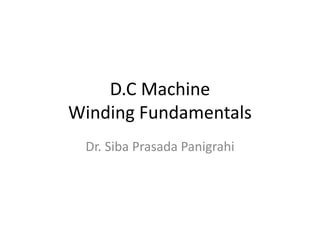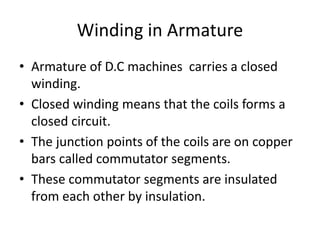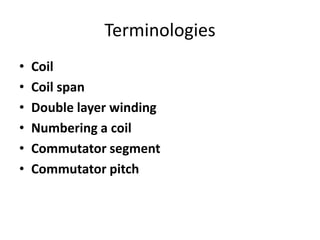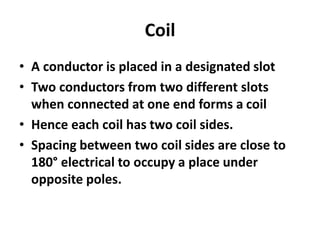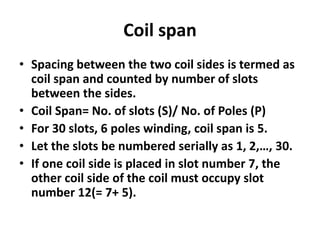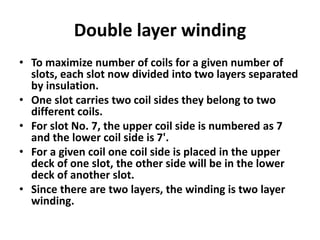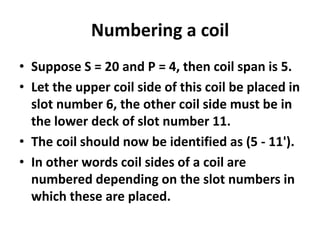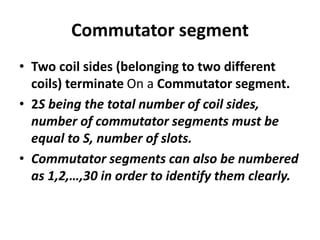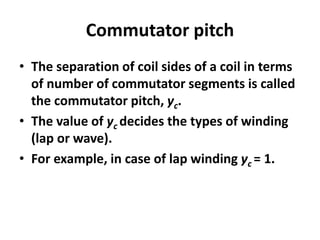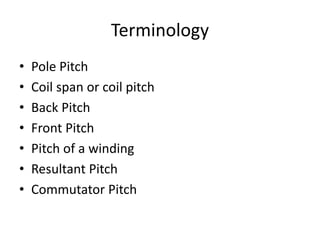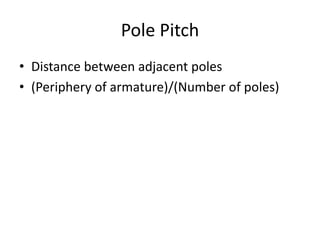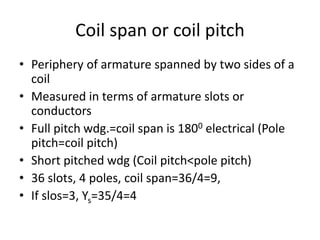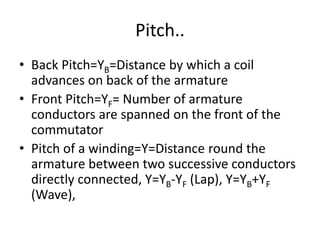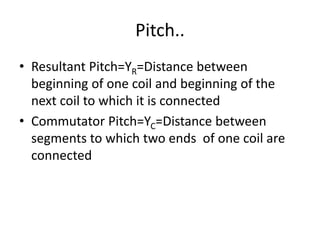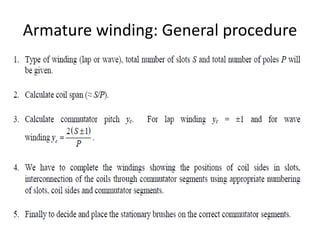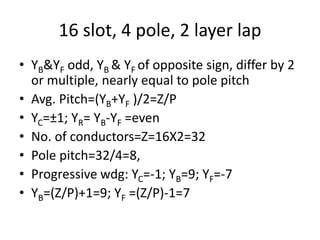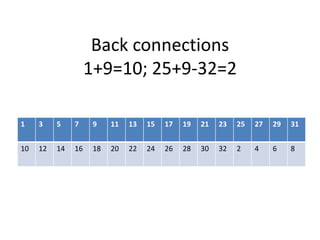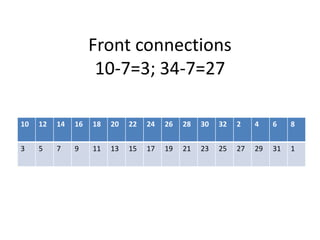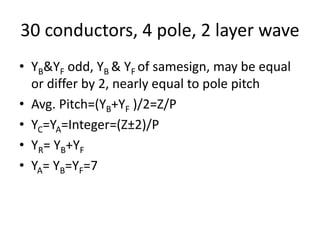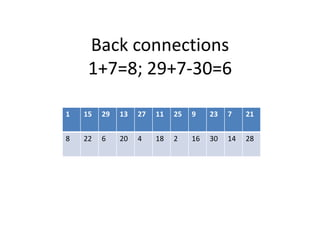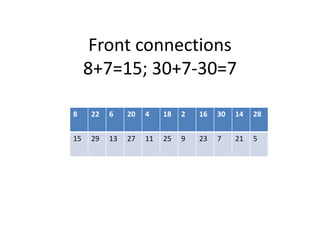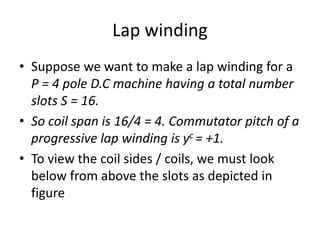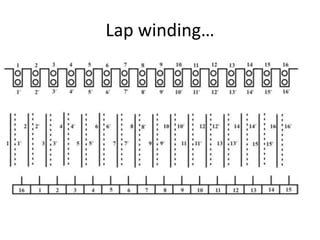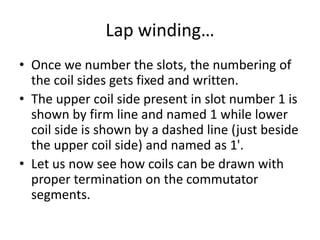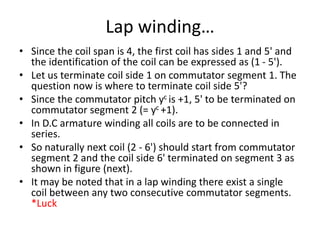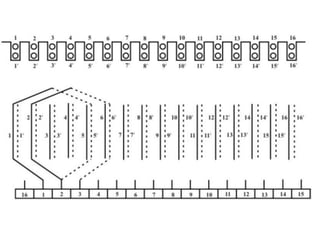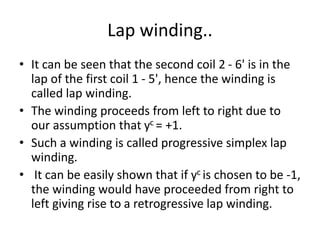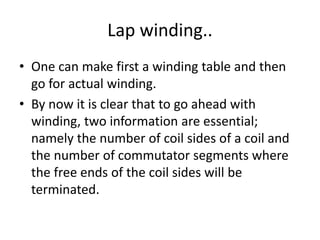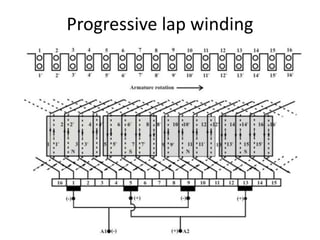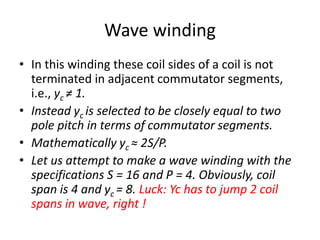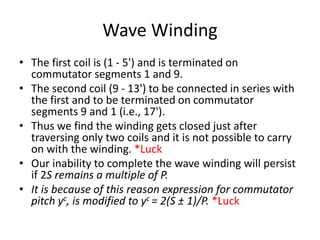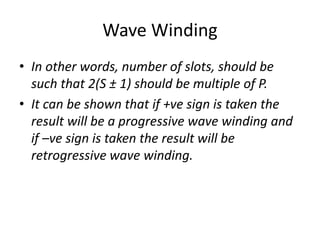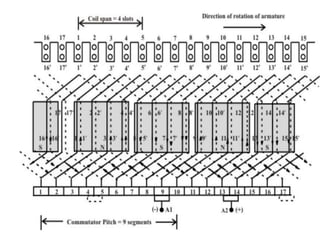1 von 34
Anzeige

1. D.C Machine Winding Fundamentals Dr. Siba Prasada Panigrahi
2. Winding in Armature • Armature of D.C machines carries a closed winding. • Closed winding means that the coils forms a closed circuit. • The junction points of the coils are on copper bars called commutator segments. • These commutator segments are insulated from each other by insulation.
3. Terminologies • Coil • Coil span • Double layer winding • Numbering a coil • Commutator segment • Commutator pitch
4. Coil • A conductor is placed in a designated slot • Two conductors from two different slots when connected at one end forms a coil • Hence each coil has two coil sides. • Spacing between two coil sides are close to 180° electrical to occupy a place under opposite poles.
5. Coil span • Spacing between the two coil sides is termed as coil span and counted by number of slots between the sides. • Coil Span= No. of slots (S)/ No. of Poles (P) • For 30 slots, 6 poles winding, coil span is 5. • Let the slots be numbered serially as 1, 2,…, 30. • If one coil side is placed in slot number 7, the other coil side of the coil must occupy slot number 12(= 7+ 5).
6. Double layer winding • To maximize number of coils for a given number of slots, each slot now divided into two layers separated by insulation. • One slot carries two coil sides they belong to two different coils. • For slot No. 7, the upper coil side is numbered as 7 and the lower coil side is 7'. • For a given coil one coil side is placed in the upper deck of one slot, the other side will be in the lower deck of another slot. • Since there are two layers, the winding is two layer winding.
7. Numbering a coil • Suppose S = 20 and P = 4, then coil span is 5. • Let the upper coil side of this coil be placed in slot number 6, the other coil side must be in the lower deck of slot number 11. • The coil should now be identified as (5 - 11'). • In other words coil sides of a coil are numbered depending on the slot numbers in which these are placed.
8. Commutator segment • Two coil sides (belonging to two different coils) terminate On a Commutator segment. • 2S being the total number of coil sides, number of commutator segments must be equal to S, number of slots. • Commutator segments can also be numbered as 1,2,…,30 in order to identify them clearly.
9. Commutator pitch • The separation of coil sides of a coil in terms of number of commutator segments is called the commutator pitch, yc. • The value of yc decides the types of winding (lap or wave). • For example, in case of lap winding yc = 1.
10. Terminology • Pole Pitch • Coil span or coil pitch • Back Pitch • Front Pitch • Pitch of a winding • Resultant Pitch • Commutator Pitch
11. Pole Pitch • Distance between adjacent poles • (Periphery of armature)/(Number of poles)
12. Coil span or coil pitch • Periphery of armature spanned by two sides of a coil • Measured in terms of armature slots or conductors • Full pitch wdg.=coil span is 1800 electrical (Pole pitch=coil pitch) • Short pitched wdg (Coil pitch<pole pitch) • 36 slots, 4 poles, coil span=36/4=9, • If slos=3, Ys=35/4=4
13. Pitch.. • Back Pitch=YB=Distance by which a coil advances on back of the armature • Front Pitch=YF= Number of armature conductors are spanned on the front of the commutator • Pitch of a winding=Y=Distance round the armature between two successive conductors directly connected, Y=YB-YF (Lap), Y=YB+YF (Wave),
14. Pitch.. • Resultant Pitch=YR=Distance between beginning of one coil and beginning of the next coil to which it is connected • Commutator Pitch=YC=Distance between segments to which two ends of one coil are connected
15. Armature winding: General procedure
16. 16 slot, 4 pole, 2 layer lap • YB&YF odd, YB & YF of opposite sign, differ by 2 or multiple, nearly equal to pole pitch • Avg. Pitch=(YB+YF )/2=Z/P • YC=±1; YR= YB-YF =even • No. of conductors=Z=16X2=32 • Pole pitch=32/4=8, • Progressive wdg: YC=-1; YB=9; YF=-7 • YB=(Z/P)+1=9; YF =(Z/P)-1=7
17. Back connections 1+9=10; 25+9-32=2 1 3 5 7 9 11 13 15 17 19 21 23 25 27 29 31 10 12 14 16 18 20 22 24 26 28 30 32 2 4 6 8
18. Front connections 10-7=3; 34-7=27 10 12 14 16 18 20 22 24 26 28 30 32 2 4 6 8 3 5 7 9 11 13 15 17 19 21 23 25 27 29 31 1
19. 30 conductors, 4 pole, 2 layer wave • YB&YF odd, YB & YF of samesign, may be equal or differ by 2, nearly equal to pole pitch • Avg. Pitch=(YB+YF )/2=Z/P • YC=YA=Integer=(Z±2)/P • YR= YB+YF • YA= YB=YF=7
20. Back connections 1+7=8; 29+7-30=6 1 15 29 13 27 11 25 9 23 7 21 8 22 6 20 4 18 2 16 30 14 28
21. Front connections 8+7=15; 30+7-30=7 8 22 6 20 4 18 2 16 30 14 28 15 29 13 27 11 25 9 23 7 21 5
22. Lap winding • Suppose we want to make a lap winding for a P = 4 pole D.C machine having a total number slots S = 16. • So coil span is 16/4 = 4. Commutator pitch of a progressive lap winding is yc = +1. • To view the coil sides / coils, we must look below from above the slots as depicted in figure
23. Lap winding…
24. Lap winding… • Once we number the slots, the numbering of the coil sides gets fixed and written. • The upper coil side present in slot number 1 is shown by firm line and named 1 while lower coil side is shown by a dashed line (just beside the upper coil side) and named as 1'. • Let us now see how coils can be drawn with proper termination on the commutator segments.
25. Lap winding… • Since the coil span is 4, the first coil has sides 1 and 5' and the identification of the coil can be expressed as (1 - 5'). • Let us terminate coil side 1 on commutator segment 1. The question now is where to terminate coil side 5'? • Since the commutator pitch yc is +1, 5' to be terminated on commutator segment 2 (= yc +1). • In D.C armature winding all coils are to be connected in series. • So naturally next coil (2 - 6') should start from commutator segment 2 and the coil side 6' terminated on segment 3 as shown in figure (next). • It may be noted that in a lap winding there exist a single coil between any two consecutive commutator segments. *Luck
26. Lap winding.. • It can be seen that the second coil 2 - 6' is in the lap of the first coil 1 - 5', hence the winding is called lap winding. • The winding proceeds from left to right due to our assumption that yc = +1. • Such a winding is called progressive simplex lap winding. • It can be easily shown that if yc is chosen to be -1, the winding would have proceeded from right to left giving rise to a retrogressive lap winding.
27. Lap winding.. • One can make first a winding table and then go for actual winding. • By now it is clear that to go ahead with winding, two information are essential; namely the number of coil sides of a coil and the number of commutator segments where the free ends of the coil sides will be terminated.
28. Progressive lap winding
29. Wave winding • In this winding these coil sides of a coil is not terminated in adjacent commutator segments, i.e., yc ≠ 1. • Instead yc is selected to be closely equal to two pole pitch in terms of commutator segments. • Mathematically yc ≈ 2S/P. • Let us attempt to make a wave winding with the specifications S = 16 and P = 4. Obviously, coil span is 4 and yc = 8. Luck: Yc has to jump 2 coil spans in wave, right !
30. Wave Winding • The first coil is (1 - 5') and is terminated on commutator segments 1 and 9. • The second coil (9 - 13') to be connected in series with the first and to be terminated on commutator segments 9 and 1 (i.e., 17'). • Thus we find the winding gets closed just after traversing only two coils and it is not possible to carry on with the winding. *Luck • Our inability to complete the wave winding will persist if 2S remains a multiple of P. • It is because of this reason expression for commutator pitch yc, is modified to yc = 2(S ± 1)/P. *Luck
31. Wave Winding • In other words, number of slots, should be such that 2(S ± 1) should be multiple of P. • It can be shown that if +ve sign is taken the result will be a progressive wave winding and if –ve sign is taken the result will be retrogressive wave winding.
32. Queries?
Anzeige